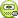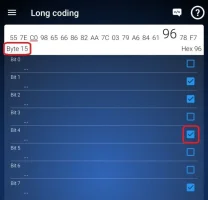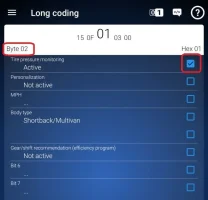# Abs Long Coding, Hill Descent Control, Tyre Pressure Monitoring

#### AJNZ

##### New Member
Here is a couple of nuggets for everyone:

How to active Tyre pressure monitoring TPM (PR 7K9)
How to active Hill descent control on 4Motion vehicles (PR UG5)

Of course with UG5 the hill descent switch must be installed and wired to the ABS CU to activate.
TPM activation is purely just software and is set/reset via the dash MFD.

T6 ABS coding: Green = Changes possible / Red = vehicle configuration / Blue = VIN serial

Byte 0 Chassis type T6 = 30, 34, 51, 55, 65 etc / Caddy = 10
Byte 1 Chassis type T6 = 7E / Caddy = 79
Byte 2 Drive train 2WD = 40 / 4WD = 80
Byte 3 Chassis type (as byte 1) 7E = 98 / 79 = 9B
Byte 4 Chassis type 25, 65 = T6 4WD / 92 = Caddy 2WD, 93 = Caddy 2WD LHD, B3 = Caddy 4WD

Byte 5 VIN serial # 13 0 = 62, 1 = 63, 2 = 64, 3 = 65, 4 = 66, 5 = 67, 6 = 68, 7 = 69, 8 = 6A, 9 = 6B
Byte 6 Configuration 84 = Hill start assist UG1 / 86 = Hill descent control UG5 - Note: Change byte 6 and 14 together
Byte 7 VIN serial # 14 0 = 7E, 1 = 7F, 2 = 80, 3 = 81, 4 = 82, 5 = 83, 6 = 84, 7 = 85, 8 = 86, 9 = 87
Byte 8 Chassis type (as byte 0) 34 = 2C, 30 = 0C, 51 = 8A, 55 = AA, 65 = 86 etc
Byte 9 VIN serial # 15 0 = 75, 1 = 76, 2 = 77, 3 = 78, 4 = 79, 5 = 7A, 6 = 7B, 7 = 7C, 8 = 7D, 9 = 7E
Byte 10 Drive train type 01 = 4WD / 02 = 2WD
Byte 11 VIN serial # 16 0 = 76, 1 = 77, 2 = 78, 3 = 79, 4 = 7A, 5 = 7B, 6 = 7C, 7 = 7D, 8 = 7E, 9 = 7F
Byte 12 Chassis type (as byte 4) A4 = T6 4WD, A6 = T6 2WD / C9 = Caddy 2WD, CD = Caddy 4WD, 49 = Caddy 2WD LHD
Byte 13 VIN serial # 17 0= 82, 1 = 83, 2 = 84, 3 = 85, 4 = 86, 5 = 87, 6 = 88, 7 = 89, 8 = 8A, 9 = 8B
Byte 14 Chassis type (as byte 6) 84= 21 (Hill start assist UG1) / 86 = 61 (Hill descent control UG5) - Note: Change byte 6 and 14 together
Byte 15 Configuration A6 = Without TPM / D3 = With TPM 7K9

Byte 16 Engine 38 = CAAC+LHD, 39=CAAC+RHD, 78 = CXHA, CXEB, CXFA, CUUD, DFSB+LHD, 29 = CAYD, CAYE+RHD, 28 = CAYD+LHD
Byte 17 ACC/City brake 00 = No ACC, F5, F7 = T6 ACC / F1, F7 = Caddy ACC

#### Lar79

##### New Member
Works pefect thanks for info

#### Pauly

##### Senior Member
VCDS User
Moderator
@Lar79 whats changes did you make/what did you activate ?

#### Lar79

##### New Member
Hi i changed byte 15 mine was B i changed to D
Worked fine set in intruments.

#### Lar79

##### New Member
Forgot TPMS activated

#### mmi

##### Senior Member
VCDS User
Moderator
VIP Member
T6 Legend
A copy from another thread ((original post))- I think this is better home for this.

TPMS activation on T6 - based on a few reported mods around the Forum. However, one definitely should take extreme care when modifying ABS settings to avoid any undesired side effects. So no responsibility acceptedOBDeleven.
Control Units.
03-Brakes - Long Coding. Example from a van with factory TPMS (+ other bits: 4Motion+diff lock, ACC, Hill descent, ESC button, etc.)---> deactivating TPMS Kombi
---> Panel van Tyre pressure monitor enable.
---> tyre pressure monitor system

Based on the links above it seems that in the ABS need to tweak bits #4 and possibly also #5 as above.
E.g.
The rest should be kept as they were in the van originally to avoid any undesired side effects.

17-Dash Board - Long Coding (this might be already set like this).Last edited:
•Robert

##### Member
T6 Pro
Here is a couple of nuggets for everyone:

How to active Tyre pressure monitoring TPM (PR 7K9)
How to active Hill descent control on 4Motion vehicles (PR UG5)

Of course with UG5 the hill descent switch must be installed and wired to the ABS CU to activate.
TPM activation is purely just software and is set/reset via the dash MFD.

T6 ABS coding: Green = Changes possible / Red = vehicle configuration / Blue = VIN serial

Byte 0 Chassis type T6 = 30, 34, 51, 55, 65 etc / Caddy = 10
Byte 1 Chassis type T6 = 7E / Caddy = 79
Byte 2 Drive train 2WD = 40 / 4WD = 80
Byte 3 Chassis type (as byte 1) 7E = 98 / 79 = 9B
Byte 4 Chassis type 25, 65 = T6 4WD / 92 = Caddy 2WD, 93 = Caddy 2WD LHD, B3 = Caddy 4WD

Byte 5 VIN serial # 13 0 = 62, 1 = 63, 2 = 64, 3 = 65, 4 = 66, 5 = 67, 6 = 68, 7 = 69, 8 = 6A, 9 = 6B
Byte 6 Configuration 84 = Hill start assist UG1 / 86 = Hill descent control UG5 - Note: Change byte 6 and 14 together
Byte 7 VIN serial # 14 0 = 7E, 1 = 7F, 2 = 80, 3 = 81, 4 = 82, 5 = 83, 6 = 84, 7 = 85, 8 = 86, 9 = 87
Byte 8 Chassis type (as byte 0) 34 = 2C, 30 = 0C, 51 = 8A, 55 = AA, 65 = 86 etc
Byte 9 VIN serial # 15 0 = 75, 1 = 76, 2 = 77, 3 = 78, 4 = 79, 5 = 7A, 6 = 7B, 7 = 7C, 8 = 7D, 9 = 7E
Byte 10 Drive train type 01 = 4WD / 02 = 2WD
Byte 11 VIN serial # 16 0 = 76, 1 = 77, 2 = 78, 3 = 79, 4 = 7A, 5 = 7B, 6 = 7C, 7 = 7D, 8 = 7E, 9 = 7F
Byte 12 Chassis type (as byte 4) A4 = T6 4WD, A6 = T6 2WD / C9 = Caddy 2WD, CD = Caddy 4WD, 49 = Caddy 2WD LHD
Byte 13 VIN serial # 17 0= 82, 1 = 83, 2 = 84, 3 = 85, 4 = 86, 5 = 87, 6 = 88, 7 = 89, 8 = 8A, 9 = 8B
Byte 14 Chassis type (as byte 6) 84= 21 (Hill start assist UG1) / 86 = 61 (Hill descent control UG5) - Note: Change byte 6 and 14 together
Byte 15 Configuration A6 = Without TPM / D3 = With TPM 7K9

Byte 16 Engine 38 = CAAC+LHD, 39=CAAC+RHD, 78 = CXHA, CXEB, CXFA, CUUD, DFSB+LHD, 29 = CAYD, CAYE+RHD, 28 = CAYD+LHD
Byte 17 ACC/City brake 00 = No ACC, F5, F7 = T6 ACC / F1, F7 = Caddy ACC

HAVE YOU ANY INFO ON THE WIRING FOR THE BUTTON??

#### Insert Coin

##### Senior Member
VIP Member
T6 Legend
HAVE YOU ANY INFO ON THE WIRING FOR THE BUTTON??
You need to mention them so they get a notification @mmi also @Robert two very clever guys amongst many on here.

•Robert

VCDS User